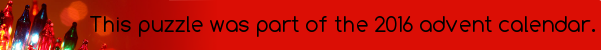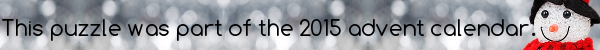mscroggs.co.ukmscroggs.co.uksubscribe

# Puzzles

## 22 December22 is two times an odd number. Today's number is the mean of all the answers on days (including today) that are two times an odd number.
Clarification: You are taking the mean for answers on days that are two times an odd numbers; ie. the days are two times odd, not the answers.

## 21 DecemberToday's number is a multiple of three. The average (mean) of all the answers that are multiples of three is a multiple of 193.
Tags: averages, mean

## 16 DecemberToday's number is four thirds of the average (mean) of the answers for 13th, 14th, 15th and 16th December.

## Mean, median, mode, range

A Find five one-digit positive integers which have a mean of 4, mode of 6, median of 4 and a range of 5.
B Find five one-digit positive integers which have a mean of 3, mode of 1, median of 1 and a range of 8.
C Find five one-digit positive integers which have a mean of 3, mode of 2, median of 2 and a range of 5.

## Archive

Show me a random puzzle
▼ show ▼